Skip to content

# marcomusy/vtkplotter

Latest commit 164008e Aug 19, 2019
Type Name Latest commit message Commit time
..
Failed to load latest commit information.advanced Aug 19, 2019basic Aug 19, 2019notebooks Aug 19, 2019other Aug 19, 2019simulations Aug 19, 2019volumetric Aug 19, 2019README.md May 16, 2019data Jun 17, 2019run_all.sh Aug 13, 2019tutorial.ipynb Aug 13, 2019tutorial.py Aug 19, 2019

# Example directories

Check out more examples in the above directories.

## Get Started tutorial

Download the tutorial:

```git clone https://github.com/marcomusy/vtkplotter.git
cd vtkplotter/examples
python tutorial.py  # on mac OSX try 'pythonw' instead```

The content of the python script and its output is the following:

```from random import gauss, uniform as u
from vtkplotter import *

# Declare an instance of the class
vp = Plotter(title='first example')

# Load a vtk file as a Actor(vtkActor) and visualize it.
# (The actual mesh corresponds to the outer shape of
# an embryonic mouse limb at about 11 days of gestation).
# Choose a tomato color for the internal surface of the mesh.
vp.load(datadir+"270.vtk").c("aqua").bc("tomato")
vp.show()  # picks what is automatically stored in python list vp.actors
# Press Esc to close the window and exit python session, or q to continue```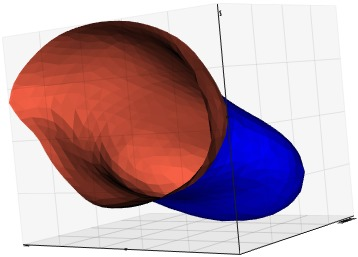```# Load 3 actors assigning each a different color,
# by default use their file names as legend entries.
# No need to use any variables, as actors are stored internally in vp.actors:
vp = Plotter(title='3 shapes')
vp.load(datadir+'250.vtk', c=(1,0.4,0), alpha=0.3) # set opacity to 30%
vp.load(datadir+'270.vtk', c=(1,0.6,0), alpha=0.3)
vp.load(datadir+'290.vtk', c=(1,0.8,0), alpha=0.3)
print('Loaded vtkActors: ', len(vp.actors))
vp.show()```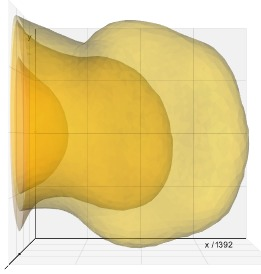```# Draw splines through a set of points, with different smoothing factors:
vp = Plotter(title='Example of splines through random points')

pts = [ (u(0,2), u(0,2), u(0,2)+i) for i in range(8) ] # build python list of points
vp.points(pts, legend='random points')                 # create the Actor

for i in range(10):
sp = spline(pts, smooth=i/10, degree=2, c=i, legend='smoothing '+str(i/10))
vp.add(sp) # add the actor to the internal list of actors to be shown
vp.show(viewup='z', interactive=1)```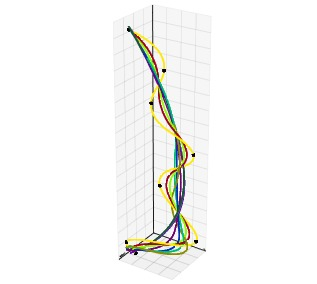```# Draw a cloud of points each point with a different color
# which depends on the point position itself
vp = Plotter(title='color points')

rgb = [(u(0,255), u(0,255), u(0,255)) for i in range(5000)]

vp.points(rgb, c=rgb, alpha=0.7, legend='RGB points')
vp.show()```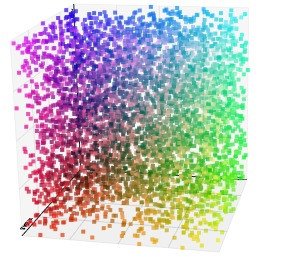```# Draw a bunch of simple objects on separate parts of the rendering window:
# split window automatically to best accomodate 9 renderers
vp = Plotter(N=9, title='basic shapes', axes=0) # split window in 9 frames
vp.sharecam = False                             # each object can be moved independently
vp.show(Arrow([0, 0, 0], [1, 1, 1]), at=0,    legend='arrow')
vp.show(Line([0, 0, 0], [1, 1, 1]), at=1,     legend='line')
vp.show(Points([[0, 0, 0], [1, 1, 1]]), at=2, legend='points')
vp.show(Text('Hello!', pos=(0, 0, 0)), at=3)
vp.show(Sphere(), at=4)
vp.show(Cube(), at=5,     legend='cube')
vp.show(Torus(), at=6,    legend='torus')
vp.show(Spring(), at=7,   legend='helix')
vp.show(Cylinder(), at=8, legend='cylinder', interactive=1)```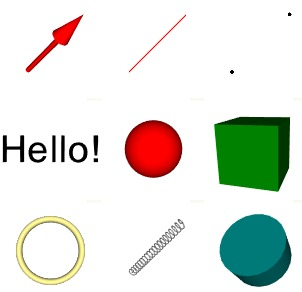```# Draw a bunch of objects from various mesh formats. Loading is automatic.
vp = Plotter(shape=(3,3), title='mesh formats') # split window in 3 rows and 3 columns
vp.sharecam = False                             # each object can be moved independently
vp.show(datadir+'beethoven.ply', at=0, c=0, axes=0)    # dont show axes
vp.show(datadir+'cow.byu',       at=1, c=1, zoom=1.15) # make it 15% bigger
vp.show(datadir+'limb.pcd',      at=2, c=2)
vp.show(datadir+'ring.gmsh',     at=3, c=3)            # show mesh as wireframe
vp.show(datadir+'images/dog.jpg',at=4)                 # 2d images can be loaded the same way
vp.show(datadir+'shuttle.obj',   at=5, c=5)
vp.show(datadir+'shapes/man.vtk',at=6, c=6, axes=2)    # show negative axes segments
vp.show(datadir+'teapot.xyz',    at=7, c=7, axes=3)    # hide negative axes
vp.show(datadir+'pulley.vtu',    at=8, c=8, interactive=1) # try to click object and press k```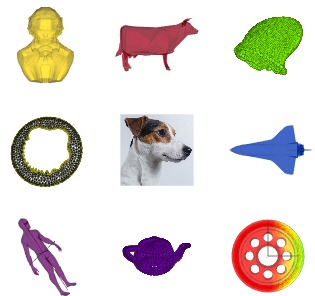```# Increase the number of vertices of a mesh using subdivide().
# Show the mesh before and after in two separate renderers defined by shape=(1,2)
vp = Plotter(shape=(1,2), axes=0) # dont show axes
a1 = vp.load(datadir+'beethoven.ply')

coords1 = a1.coordinates() # get coordinates of mesh vertices
pts1 = vp.points(coords1, r=4, c='g', legend='#points = '+str(len(coords1)))
vp.show([a1, pts1], at=0) # show a specific list of actors on renderer nr.0

a2 = a1.subdivide() # Increase the number of points of the mesh
coords2 = a2.coordinates()
pts2 = vp.points(coords2, r=1, legend='#points = '+str(len(coords2)))
vp.show([a2, pts2], at=1, interactive=True)```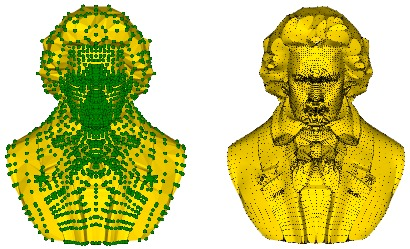```#########################################################################################
# Cut a set of shapes with a plane that goes through the
# point at x=500 and has normal (0, 0.3, -1).
# Wildcards can be used to load multiple files or entire directories:
vp = Plotter(title='Cut a surface with a plane')
vp.load(datadir+'2*0.vtk')
for a in vp.actors:
a.c("orange").bc("aqua")
a.cutWithPlane(origin=(500,0,0), normal=(0,0.3,-1), showcut=True)
vp.show()```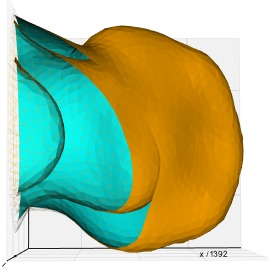### Some useful Plotter attributes

Remember that you always have full access to all standard VTK native objects (e.g. vtkRenderWindowInteractor, vtkRenderer and vtkActor through vp.interactor, vp.renderer, vp.actors... etc).

```vp = vtkplotter.Plotter() #e.g.
vp.actors       # holds the current list of vtkActors to be shown
vp.renderer     # holds the current vtkRenderer
vp.renderers    # holds the list of renderers
vp.interactor   # holds the vtkWindowInteractor object
vp.interactive  # (True) allows to interact with renderer after show()
vp.camera       # holds the current vtkCamera object
vp.sharecam     # (True) share the same camera in multiple renderers```

### Some useful additional methods to manage 3D objects

These methods return the Actor(vtkActor) object so that they can be concatenated, check out Actor methods here.
(E.g.: `actor.scale(3).pos([1,2,3]).color('blue').alpha(0.5)` etc..).

```actor.pos()               # set/get position vector (setters, and getters if no argument is given)
actor.scale()             # set/get scaling factor of actor
actor.normalize()         # sets actor at origin and scales its average size to 1
actor.rotate(angle, axis) # rotate actor around axis
actor.color(name)         # sets/gets color
actor.alpha(value)        # sets/gets opacity
actor.N()                 # get number of vertex points defining the actor's mesh
actor.polydata()          # get the actor's mesh vtkPolyData in its current transformation
actor.coordinates()       # get a copy of vertex points coordinates (copy=False to get references)
actor.clone()             # generate a copy of actor
...```

### Available color maps from matplotlib and vtkNamedColors

```# Example: transform a scalar value between -10.2 and 123 into a (R,G,B) color using the 'jet' map:
r, g, b = colorMap(value, name='jet', vmin=-10.2, vmax=123)```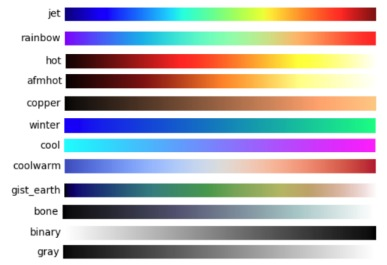A list of available vtk color names is given here.

You can’t perform that action at this time.